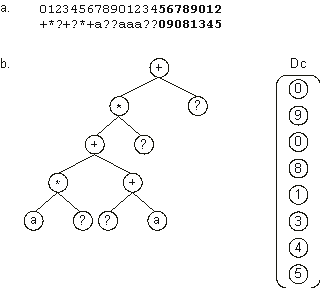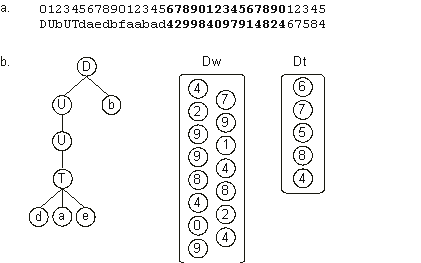Home
News
Author
Q&A
Tutorials
GEP Biblio
Contacts

Visit Gepsoft

Gene Expression Programming: Mathematical Modeling by an Artificial Intelligence

Other levels of complexity

As we have seen in the examples above, although a very simple system, gene expression programming exhibits already a complex development. The most complex individuals evolved by GEP contain, besides the head and the tail, extra domains encoding one-element sub-ETs. These one-element sub-ETs interact with the main sub-ET encoded in the head/tail domain, forming a more complex entity with a complex network of interactions.

One such architecture was developed to manipulate random numerical constants in symbolic regression (Ferreira 2001). For instance, the following chromosome:

 01234567890123456789012 +*?+?*+a??aaa??09081345 (2.28)

contains an extra domain Dc (shown in blue) encoding random numerical constants. Its translation is shown in Figure 2.14. In section 4.2 we will learn how these sub-ETs interact with one another so that the individual is fully developed.Figure 2.14. Translation of chromosomes with multiple domains. a) A multi-domain chromosome composed of a conventional head/tail domain and an extra domain (Dc) encoding random numerical constants (shown in bold). b) The sub-ETs codified by each gene. The one-element sub-ETs encoded in Dc are placed together apart. “?” represents the random numerical constants encoded in the numerals of Dc. How all these sub-ETs interact will be shown in section 4.2.

Multiple domains are also used to design neural networks totally encoded in a linear genome (see chapter 5). These neural networks are one of the most complex kind of individual evolved by GEP. In this case, the network architecture is encoded in a conventional head/tail domain whereas the weights and thresholds are encoded in two extra domains, Dw and Dt, each composed of one-element genes. The chromosome below contains two extra domains encoding the weights and the thresholds (the domains are shown in different colors):